Logarithmic Regression Model Example

Data:  The data below show the average growth rates of 12 Weeping Higan cherry trees planted in Washington, D.C.  At the time of planting, the trees were one year old and were all 6 feet in height.Age of Tree
(in years)
Height
(in feet)
1
6
2
9.5
3
13
4
15
5
16.5
6
17.5
7
18.5
8
19
9
19.5
10
19.7
11
19.8

 Task: a.) Determine a logarithmic regression model equation to represent this data. b.) Graph the new equation. c.) Decide whether the new equation is a "good fit" to represent this data. d.) Interpolate:  What was the average height of the trees at one and one-half years of age?  (to the nearest tenth of a foot) e.) Extrapolate:  What is the predicted average height of the trees at 20 years of age?Is this prediction realistic?  (answer to the nearest tenth of a foot) f.) Based upon your observations of this data, what would you predict to be the average height of a mature Higan cherry tree, to the nearest foot? g.) If the average height of the trees is 10 feet, what is the age of the trees to the nearest tenth of a year?
 Step 1.  Enter the data into the lists.  For basic entry of data, see Basic Commands.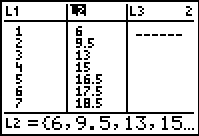Step 2.  Create a scatter plot of the data.  Go to STATPLOT (2nd Y=) and choose the first plot.  Turn the plot ON, set the icon to Scatter Plot (the first one), set Xlist to L1 and Ylist to L2 (assuming that is where you stored the data), and select a Mark of your choice.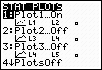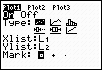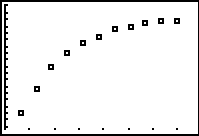Step 3.  Choose Logarithmic Regression Model. Press STAT, arrow right to CALC, and arrow down to 9: LnReg.  Hit ENTER.  When LnReg appears on the home screen, type the parameters L1, L2, Y1.  The Y1 will put the equation into Y= for you.  (Y1 comes from VARS → YVARS, #Function, Y1)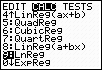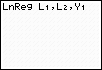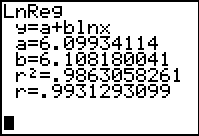The logarithmic regression equation is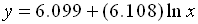(answer to part a) Step 4.  Graph the Exponential Regression Equation from Y1. ZOOM #9 ZoomStat to see the graph.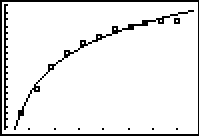(answer to part b) Step 5.  Is this model a "good fit"? The correlation coefficient, r, is .9931293099 which places the correlation into the "strong" category.  (0.8 or greater is a "strong" correlation) The coefficient of determination, r 2, is .9863058261 which means that 98.6% of the total variation in y can be explained by the relationship between x and y.       Yes, it is a very "good fit".          (answer to part c)Step 6.  Interpolate: (within the data set)       What was the average height of the trees at one and one-half years of age?  (to the nearest tenth of a foot) The logarithmic regression equation iswhere x stands for time.  Substituting 1.5 for x gives an average height of 8.57558088 feet or 8.6 feet. (answer to part d) Step 7. Extrapolate data: (beyond data set)    What is the predicted average height of the trees at 20 years of age?  Is this prediction realistic?  (answer to the nearest tenth of a foot)    The logarithmic regression equation iswhere x stands for time.  Substituting 20 for x gives an average height of 24.39693273 feet or 24.4 feet. Extrapolations far from the stated data are often inaccurate and unreliable.  Nine years away from the data set is a large span of time and the reading of 24.4 feet may be "high" based upon the observed leveling nature of the last data entries. (answer to part e) Step 8.      Based upon your observations of this data, what would you predict to be the average height of a mature Higan cherry tree, to the nearest foot? It can be seen from the graph that the growth rate is slowing down (leveling off).  Such a slowing could be interpreted that the trees are reaching their mature height.  The twenty year prediction shows a height of 24 feet.  Any answer from 20 feet to 24 feet would be an acceptable approximation.  While extrapolations far from the stated data are often inaccurate, a reading closer to 20 feet may be more accurate. (In reality, Higan cherry trees, depending upon the specific specie, reach a mature height anywhere from 15 to 30 feet.) (answer to part f) Step 9.   If the average height of the tree is 10 feet, what is the age of the tree to the nearest tenth of a year? Use your table to find the age:(answer to part g -- approx. 1.9 years of age)Finding Your Way Around TABLE of  CONTENTS